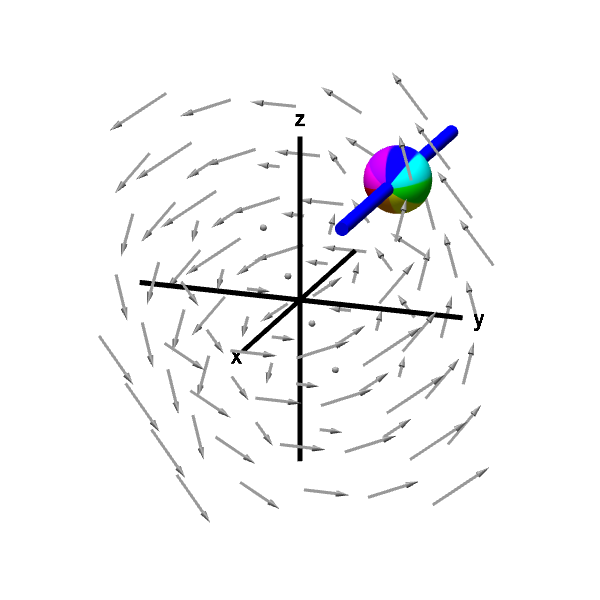# Math Insight

### Applet: A rotating sphere on a rod gives x-component of curlThe rod constrains the rotation of the sphere to point (using the right hand rule) in either the positive or negative $x$ direction. The speed of this rotation corresponds to the $x$-component of the curl. In this case, the rotation is counterclockwise when viewed from the positive $x$-axis, corresponding to a positive $x$-component of the curl. In this case, the rotation is counterclockwise when viewed from the positive $x$-axis, corresponding to a positive $x$-component of the curl.

This vector field is $\dlvf(x,y,z)= (-y,x-z,y).$ Its curl is \begin{align*} \curl \dlvf(x,y,z) &= \left(\pdiff{}{y}y-\pdiff{}{z}(x-z), \pdiff{}{z}(-y)- \pdiff{}{x}y, \pdiff{}{x}(x-z) - \pdiff{}{y}(-y) \right)\\ &=(2,0,2). \end{align*} In particular, the $x$-component corresponding to the sphere's rotation is 2. Since the $x$-component of the curl is constant, the rotation of the sphere does not change if you move the sphere.